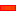﻿ Epitrochoid

# Epitrochoid

## Epicycloid and Epitrochoid Curves

 A point on the pitch-circle of a gear that rolls around the outside of a stationary gear gives a curve called an Epicycloid. If the point is not on the pitch-circle of the rolling gear, it has a generic name of Epitrochoid. If the point is inside or outside the pitch-circle: Curtate and Prolate Epitrochoid Curves are traced by a Point that is on a gear that rolls (orbits) around the outside of a fixed internal gear. The shape of the curve depends on the ratio of the teeth. The ratio of the teeth is always a rational number. Therefore, the curve repeats, even if the gear ratio is a ratio of two prime numbers.Equal Number of Gear Teeth. This curve is called a Limaҫon. If the Point is on the Pitch-Circle it is called a Cardioid. The image shows four curves. Epicycloid One curveis given by a point on the pitch-circle of the orbiting gear - it is the Epicycloid Epitrochoid Two Protracted Epitrochoid curvesare given by points outside the pitch-circle. One Contracted Epitrochoid curvesis given by a point inside the pitch-circle.The External Gear is half the diameter of the fixed gear. This curve is called a Nephroid. The image shows four curves. Epicycloid One curve is given by a point on the pitch-circle - it is the Epicycloid Epitrochoid Two curves are given by points outside the pitch-circle One curve is given by a point inside the pitch-circleThe Gear ratio is 8: 1 There are four curves: 2 outside, one inside, one on the pitch-circleGear Ratio is 13:11 There is ONE curve. Use a Gearing FB to rotate the carrier 11 times to complete the Epicycloid Path.Gear Ratio 2:3 There is ONE Curve. Use a Gearing FB to rotate the carrier 3 times to complete the Epicycloid Path.Gear Ratio 24:6 There is ONE Curve. This is because the ratio reduces to 4:1Epicycloid and the Hypocycloid Curves that give Straight-Lines

 One of the most basic Epicycloid and Hypocycloid Curves that the Gear-Pairs can give is a perfect Straight Line. Please see YouTube video to show designs.Straight Line Curve with Hypocycloid Gears - Internal Mesh The Video shows the Trace-Point follows a perfectly straight line. You can see that the smaller gear has half the teeth of the other. This is the standard Straight-Line Epicyclic Gear-Pair configuration.Straight Line Curve with Epicycloid Gears - External Mesh - 1 - the Easy Way There are two ways to assemble this mechanism in MechDesigner The not easy, and the easy way. This is the Easy WayStraight Line Curve with Epicycloid Gears - External Mesh - 2 - the Not Easy Way   Spot the difference.

Tutorial and Reference Help Files for MechDesigner and MotionDesigner 13.2 + © Machine, Mechanism, Motion and Cam Design Software by PSMotion Ltd